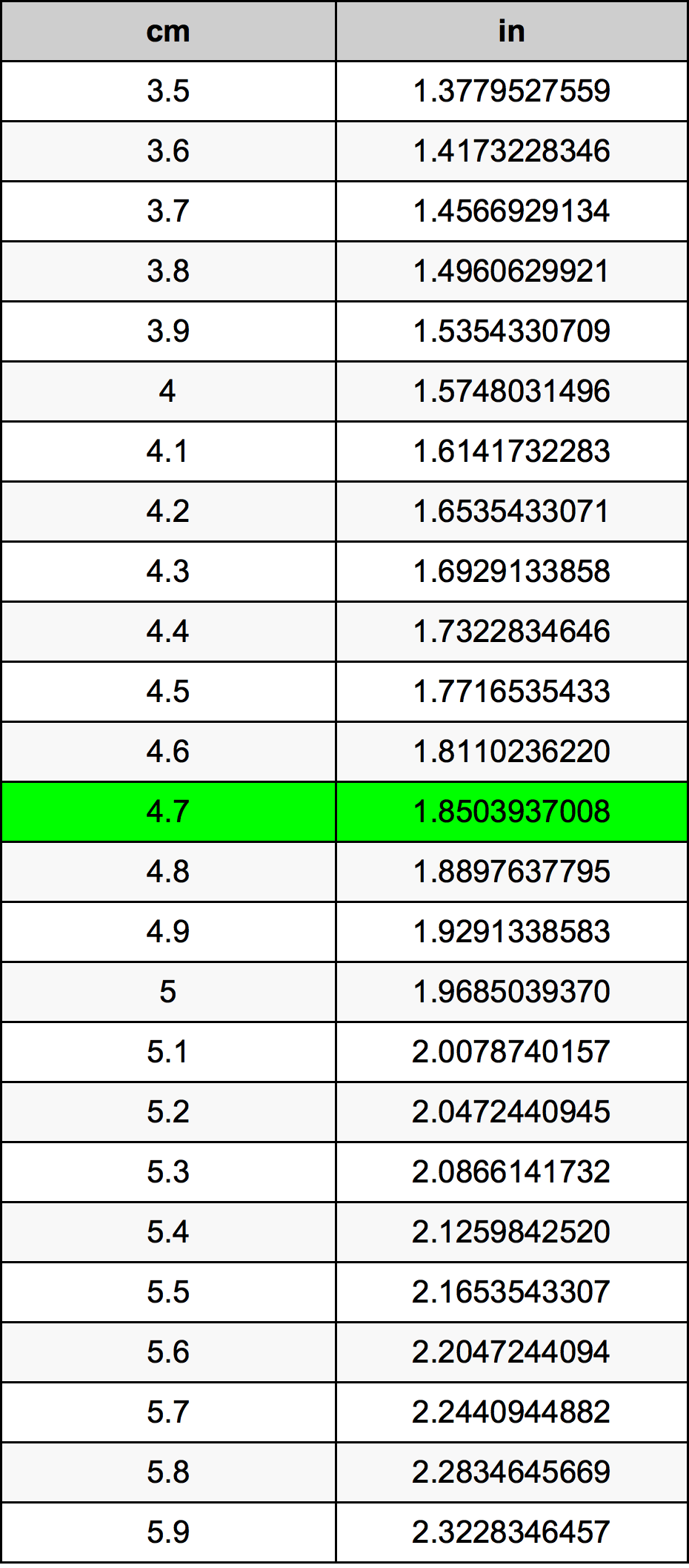Cm To Inches

# 4.7 cm to in4.7 Centimeters to Inches

cm
=
in

## How to convert 4.7 centimeters to inches?

 4.7 cm * 0.3937007874 in = 1.8503937008 in 1 cm
A common question is How many centimeter in 4.7 inch? And the answer is 11.938 cm in 4.7 in. Likewise the question how many inch in 4.7 centimeter has the answer of 1.8503937008 in in 4.7 cm.

## How much are 4.7 centimeters in inches?

4.7 centimeters equal 1.8503937008 inches (4.7cm = 1.8503937008in). Converting 4.7 cm to in is easy. Simply use our calculator above, or apply the formula to change the length 4.7 cm to in.

## Convert 4.7 cm to common lengths

UnitUnit of length
Nanometer47000000.0 nm
Micrometer47000.0 µm
Millimeter47.0 mm
Centimeter4.7 cm
Inch1.8503937008 in
Foot0.1541994751 ft
Yard0.051399825 yd
Meter0.047 m
Kilometer4.7e-05 km
Mile2.92044e-05 mi
Nautical mile2.5378e-05 nmi

## What is 4.7 centimeters in in?

To convert 4.7 cm to in multiply the length in centimeters by 0.3937007874. The 4.7 cm in in formula is [in] = 4.7 * 0.3937007874. Thus, for 4.7 centimeters in inch we get 1.8503937008 in.

## 4.7 Centimeter Conversion Table## Alternative spelling

4.7 cm to in, 4.7 cm in in, 4.7 Centimeter to in, 4.7 Centimeter in in, 4.7 Centimeter to Inches, 4.7 Centimeter in Inches, 4.7 Centimeter to Inch, 4.7 Centimeter in Inch, 4.7 cm to Inch, 4.7 cm in Inch, 4.7 Centimeters to Inch, 4.7 Centimeters in Inch, 4.7 cm to Inches, 4.7 cm in Inches